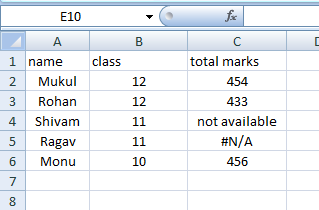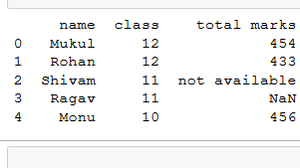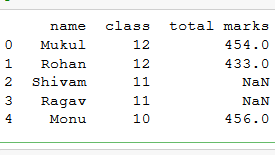# Use of na_values parameter in read_csv() function of Pandas in Python

• Difficulty Level : Basic
• Last Updated : 28 Jul, 2020

read_csv() is an important pandas function to read CSV files. But there are many other things one can do through this function only to change the returned object completely. In this post, we will see the use of the na_values parameter.

na_values: This is used to create a string that considers pandas as NaN (Not a Number). by-default pandas consider #N/A, -NaN, -n/a, N/A, NULL etc as NaN value. let’s see the example for better understanding.so this is our dataframe it has three column names, class, and total marks. now import the dataframe in python pandas.

Refer the link to the data set used from here.

Example 1: see pandas consider #N/A as NaN.

## Python3

 `# import pandas library``import` `pandas as pd`` ` `# read a csv file``df ``=` `pd.read_csv(``'Example.csv'``)`` ` `# show the dataframe``print``(df)`

Output:Example 2: Now the na_values parameter is used to tell pandas they consider “not available” as NaN value and print NaN at the place of “not available”.

## Python3

 `# import pandas library``import` `pandas as pd`` ` `# read a csv file``df ``=` `pd.read_csv(``'Example.csv'``, ``                 ``na_values ``=` `"not available"``)`` ` `# show the dataframe``print``(df)`

Output:My Personal Notes arrow_drop_up